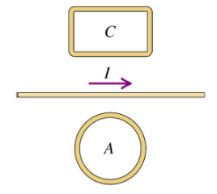# Problem: Two closed loops A and C are close to a straight wire carrying a current I.(a) Find the direction (clockwise or counterclockwise) of the current induced in the loop C if I is steadily increasinga. The current in loop C is counterclockwiseb. The current in loop C is clockwisec. The current in loop C is zero(b) While I is increasing, what is the direction of the net force that the wire exerts on loop C?a. The wire exerts an upward force on loop Cb. The wire exerts a downward force on loop Cc. The wire exerts no force on loop C

###### FREE Expert Solution

Right-hand rule for straight conductors: Thumb → current, fingers → curl in the direction of the magnetic field.

Right-hand rule for loops: Thumb → magnetic field. Fingers → curl in the direction of the current.

Lenz's law:

The induced current creates an induced magnetic field that opposes the change in magnetic flux inducing it.

(a)

The field due to the wire carrying a current in loop C is out of the page. The change in flux is increasing.

The induced field is into the page to oppose the increasing flux.

79% (309 ratings)###### Problem Details

Two closed loops A and C are close to a straight wire carrying a current I.(a) Find the direction (clockwise or counterclockwise) of the current induced in the loop C if I is steadily increasing

a. The current in loop C is counterclockwise

b. The current in loop C is clockwise

c. The current in loop C is zero

(b) While I is increasing, what is the direction of the net force that the wire exerts on loop C?

a. The wire exerts an upward force on loop C

b. The wire exerts a downward force on loop C

c. The wire exerts no force on loop C